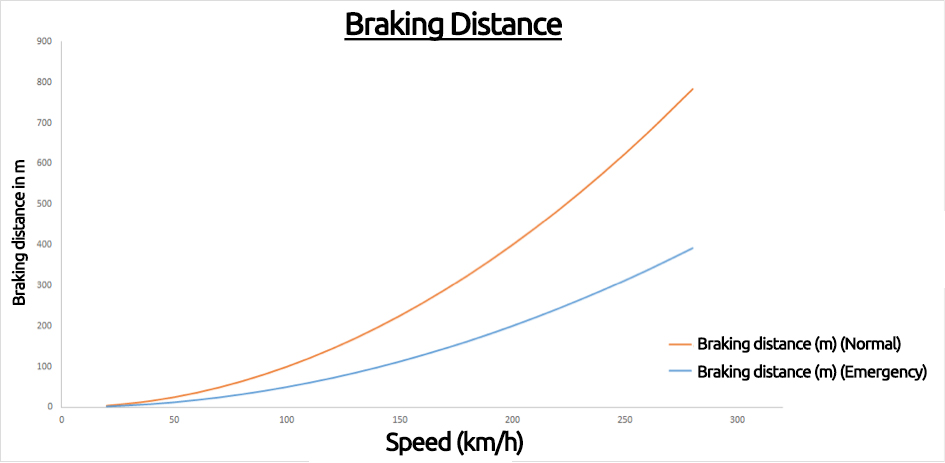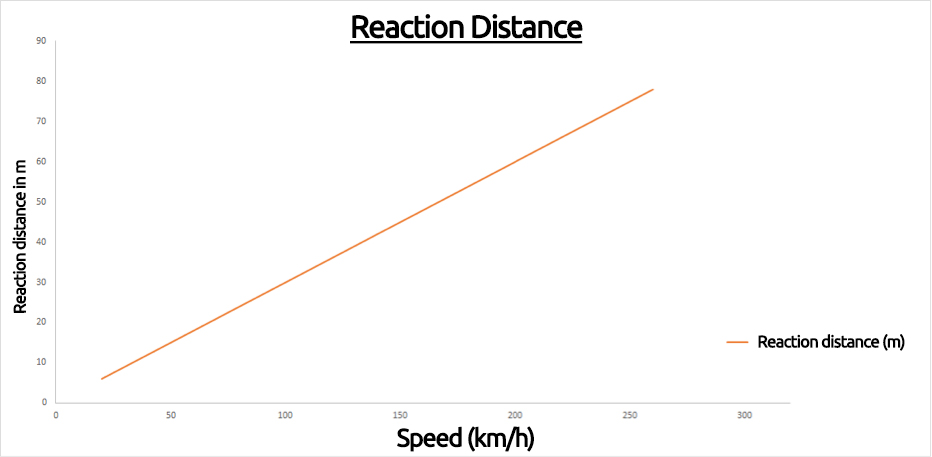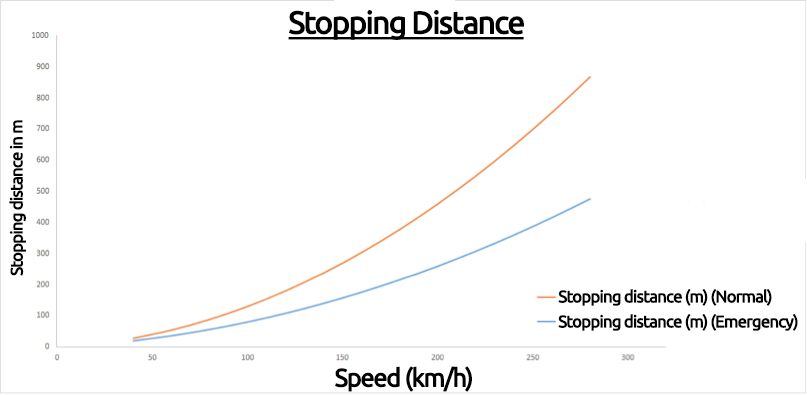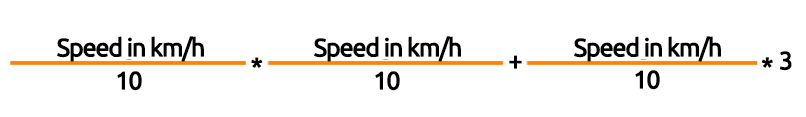Braking distance, reaction distance, stopping distance

When the car comes to a standstill

Every year there are thousands of traffic accidents on UAE roads. Frequently, the reaction time or the actual braking distance was underestimated.

Braking Distance Calculator gives the measure of braking distance required at various speeds to avoid serious accidents. Drivers have learnt the meaning of these variables in driving school, but the calculation thereof remains a mystery for many road users.

SellCarGetCash.com clarifies in the following article, what it means and provides you with an exclusive calculation, which determines both stopping distance, as well as reaction and braking distance calculated according to the speed.

Braking distance, braking time and emergency braking

The braking distance is the distance between the start of braking and when the vehicle stops. It states how many meters the car travelled during braking. In contrast, there is the braking time that includes the braking distance and the brake response. This depends on the speed of travel and a possible deceleration. The actual length of the braking distance depends on various factors, such as the road surface, the condition of the road, the vehicle itself and on the magnitude of which the driver brakes. Of course, the braking system’s technical system must also be intact. If this is not the case, the tires are worn or the roads are slippery, then the braking distance increases. The same applies when the braking takes place downhill. The following rule of thumb when calculating the braking distance is taught in driving schools:This calculation is based on a normal brake application without being affected by slippery roads or the like and describes the distance to stop the car. This formula is the basis of all the calculations of the theoretical test within the driving school.

Sample calculation:

If a car is moving at a speed of 120 kilometres per hour, then the braking distance is 144 meters.

Calculation: 120/10 * 120/10 = 144 meters.

Attention: With twice the speed, the braking distance is four times as much!

In contrast to the normal braking, emergency braking that is full braking, the clutch and brake are simultaneously required. The basis of calculation is the same, but the result is divided by 2. Emergency braking at 120 kilometres per hour thus gives a braking distance of 72 meters.

Braking distance table

 Speed in km/h Braking distance in m (normal) Braking distance in m (emergency) 20 km/h 4 Meter 2 Meter 30 km/h 9 Meter 4,5 Meter 40 km/h 16 Meter 8 Meter 50 km/h 25 Meter 12,5 Meter 60 km/h 36 Meter 18 Meter 80 km/h 64 Meter 32 Meter 100 km/h 100 Meter 50 Meter 120 km/h 144 Meter 72 Meter 130 km/h 169 Meter 84,5 Meter 140 km/h 196 Meter 98 Meter 160 km/h 256 Meter 128 Meter 180 km/h 324 Meter 162 Meter 200 km/h 400 Meter 200 Meter 220 km/h 484 Meter 242 Meter 240 km/h 576 Meter 288 Meter 260 km/h 676 Meter 338 Meter 280 km/h 784 Meter 392 Meter

Reaction distance

In comparison to the braking distance, the reaction distance comprises on the one hand, the reaction time required by the human body to respond to a potential hazard and secondly, the physical reaction, that is, the actual operation of the brake pedal by the driver. For this process, motorists need on average one second. However, the reaction distance is longer when the driver is under the influence of drugs, is particularly tired, has taken certain drugs or is distracted while driving. Research states that the reaction time is reduced with a blood alcohol level of 0.8 to about 50 percent. The fact that this circumstance can be highly dangerous is shown using the rule of thumb for calculating the reaction distance, which is based on the normal response time of one second:The formula indicates how many meters the driver travels within the reaction time at a certain speed.

Sample calculation:

If a car is travelling at a speed of 80 kilometres per hour, then the reaction distance at a second reaction time is 24 meters.

Calculation: 80/10 * 3 = 24 meters.

Motorists react slower under the influence of alcohol, the time increases to at least two seconds, which doubles the reaction distance. For a car with a speed of 80 kilometres per hour, this represents a reaction distance of 48 meters.

Reaction distance table

 Speed in km/h Reaction distance in m 20 km/h 6 Meter 30 km/h 9 Meter 40 km/h 12 Meter 50 km/h 15 Meter 60 km/h 18 Meter 80 km/h 24 Meter 100 km/h 30 Meter 120 km/h 36 Meter 130 km/h 39 Meter 140 km/h 42 Meter 160 km/h 48 Meter 180 km/h 54 Meter 200 km/h 60 Meter 220 km/h 66 Meter 240 km/h 72 Meter 260 km/h 78 Meter

Stopping distance

The stopping distance is composed of the reaction distance and the braking distance. That means that it begins with recognizing the danger and ends when the vehicle is stopped:The stopping distance depends on the driver (responsiveness), the conditions of the road and the braking, so you cannot individually calculate it. To calculate the stopping distance the rule of thumb is:It is composed of the calculation of the reaction and braking distance. Both results are added. If there is an emergency braking, the stopping distance must be divided by 2 when calculating the braking distance.

Sample calculation:

If the car is travelling at a speed of 120km / h, the entire stopping distance is 180m.

Calculation: 120/10 * 120/10 + 120/10 * 3 = 180 meters.

Exception: If it is emergency braking, it is reduced to 108m.

Stopping distance table

 Speed in km/h Stopping distance in m (normal) Stopping distance in m (emergency) 20 km/h 10 Meter 8 Meter 30 km/h 18 Meter 13,5 Meter 40 km/h 28 Meter 20 Meter 50 km/h 40 Meter 27,5 Meter 60 km/h 54 Meter 36 Meter 80 km/h 88 Meter 56 Meter 100 km/h 130 Meter 80 Meter 120 km/h 180 Meter 108 Meter 130 km/h 208 Meter 123,5 Meter 140 km/h 238 Meter 140 Meter 160 km/h 304 Meter 176 Meter 180 km/h 378 Meter 216 Meter 200 km/h 460 Meter 260 Meter 220 km/h 550 Meter 308 Meter 240 km/h 648 Meter 360 Meter 260 km/h 754 Meter 416 Meter 280 km/h 868 Meter 476 Meter

SellCarGetCash.com provides the best car buying services in the UAE. We give the assurance of buying any car that we inspect even if it is a damaged car. We buy all used cars including the ones which have suffered from accidents and have minor or major damages. We offer best prices for all used cars that we buy.